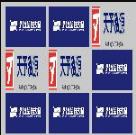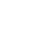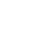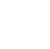• 定制开发
• APP定制开发
• 智能软硬件应用
• 戒赌难戒视角
• 技术VC
• 关于我们
• 联系我们
• 戒赌难戒视角

关注互联网，关注技术开发，透析与分享移动互联网行业最新动态
当前位置： 网站首页 > 解决方案 > php图片合成方法(多张图片合成一张)

php图片合成方法(多张图片合成一张)

时间：2019-02-18 18:24:26    阅读：555次 分类：解决方案
1.多张图片合成一张比如： 图片合成，可以显示在浏览器上面同时保存到文件夹下面 实例如下所示： ? 1 2 3 4 5 6 7 8 9 10 11 12 13 14 15 16 17 18 19 20 21 22 23 24 25 26 27 28 29 30 31 32 33 34 35 36 37 38 39 40 41 42 43 44 45 46 47 48 49 50...

1.多张图片合成一张比如：

图片合成，可以显示在浏览器上面同时保存到文件夹下面实例如下所示：

1
2
3
4
5
6
7
8
9
10
11
12
13
14
15
16
17
18
19
20
21
22
23
24
25
26
27
28
29
30
31
32
33
34
35
36
37
38
39
40
41
42
43
44
45
46
47
48
49
50
51
52
53
54
55
56
57
58
59
60
61
62
63
64
65
66
67
68
69
70
71
72
73
74
75
76
77
78
79
80
81
82
83
84
85
86
87
88
89
90
91
92
93
94
95
96
97
98
99
100
101
102
103
104
105
106
107
108
109
110
111
112
113
114
115
116
117
118
119
120
121
122
123
124
125
126
127
128
129
130
131
132
133
134
135
136
137
`<?``php`
` ``/** `
` ``* 图片合并 `
` ``**/ `
` `
` ``\$``pic_list`  `= ``array``( `
`  ``'img2.png', `
`  ``'img2.png', `
`  ``'logo.png', `
`  ``'logo.png', `
`  ``'logo.png', `
`  ``'img2.png', `
`  ``'img2.png', `
`  ``'img2.png', `
`  ``'img2.png' `
` ``); `
`  `
` ``\$``pic_list` `= ``array_slice``(\$pic_list, 0, 9); // 只操作前9个图片 `
` `
` ``\$``bg_w` `= ``150``; // 背景图片宽度 `
` ``\$``bg_h` `= ``150``; // 背景图片高度 `
` `
` ``\$``background` `= ``imagecreatetruecolor``(\$bg_w,\$bg_h); // 背景图片 `
` ``\$``color` `= ``imagecolorallocate``(\$background, 202, 201, 201); // 为真彩色画布创建白色背景，再设置为透明 `
` ``imagefill(\$background, 0, 0, \$color); `
` ``imageColorTransparent(\$background, \$color); `
` `
` ``\$``pic_count` `= count(\$pic_list); `
` ``\$``lineArr` `= ``array``(); // 需要换行的位置 `
` ``\$``space_x` `= ``3``; `
` ``\$``space_y` `= ``3``; `
` ``\$``line_x` `= ``0``; `
` ``switch(\$pic_count) { `
` ``case 1: // 正中间 `
`  ``\$``start_x` `= ``intval``(\$bg_w/4); // 开始位置X `
`  ``\$``start_y` `= ``intval``(\$bg_h/4); // 开始位置Y `
`  ``\$``pic_w` `= ``intval``(\$bg_w/2); // 宽度 `
`  ``\$``pic_h` `= ``intval``(\$bg_h/2); // 高度 `
`  ``break; `
` ``case 2: // 中间位置并排 `
`  ``\$``start_x` `= ``2``; `
`  ``\$``start_y` `= ``intval``(\$bg_h/4) + 3; `
`  ``\$``pic_w` `= ``intval``(\$bg_w/2) - 5; `
`  ``\$``pic_h` `= ``intval``(\$bg_h/2) - 5; `
`  ``\$``space_x` `= ``5``; `
`  ``break; `
` ``case 3: `
`  ``\$``start_x` `= ``40``; // 开始位置X `
`  ``\$``start_y` `= ``5``; // 开始位置Y `
`  ``\$``pic_w` `= ``intval``(\$bg_w/2) - 5; // 宽度 `
`  ``\$``pic_h` `= ``intval``(\$bg_h/2) - 5; // 高度 `
`  ``\$``lineArr` `= ``array``(2); `
`  ``\$``line_x` `= ``4``; `
`  ``break; `
` ``case 4: `
`  ``\$``start_x` `= ``4``; // 开始位置X `
`  ``\$``start_y` `= ``5``; // 开始位置Y `
`  ``\$``pic_w` `= ``intval``(\$bg_w/2) - 5; // 宽度 `
`  ``\$``pic_h` `= ``intval``(\$bg_h/2) - 5; // 高度 `
`  ``\$``lineArr` `= ``array``(3); `
`  ``\$``line_x` `= ``4``; `
`  ``break; `
` ``case 5: `
`  ``\$``start_x` `= ``30``; // 开始位置X `
`  ``\$``start_y` `= ``30``; // 开始位置Y `
`  ``\$``pic_w` `= ``intval``(\$bg_w/3) - 5; // 宽度 `
`  ``\$``pic_h` `= ``intval``(\$bg_h/3) - 5; // 高度 `
`  ``\$``lineArr` `= ``array``(3); `
`  ``\$``line_x` `= ``5``; `
`  ``break; `
` ``case 6: `
`  ``\$``start_x` `= ``5``; // 开始位置X `
`  ``\$``start_y` `= ``30``; // 开始位置Y `
`  ``\$``pic_w` `= ``intval``(\$bg_w/3) - 5; // 宽度 `
`  ``\$``pic_h` `= ``intval``(\$bg_h/3) - 5; // 高度 `
`  ``\$``lineArr` `= ``array``(4); `
`  ``\$``line_x` `= ``5``; `
`  ``break; `
` ``case 7: `
`  ``\$``start_x` `= ``53``; // 开始位置X `
`  ``\$``start_y` `= ``5``; // 开始位置Y `
`  ``\$``pic_w` `= ``intval``(\$bg_w/3) - 5; // 宽度 `
`  ``\$``pic_h` `= ``intval``(\$bg_h/3) - 5; // 高度 `
`  ``\$``lineArr` `= ``array``(2,5); `
`  ``\$``line_x` `= ``5``; `
`  ``break; `
` ``case 8: `
`  ``\$``start_x` `= ``30``; // 开始位置X `
`  ``\$``start_y` `= ``5``; // 开始位置Y `
`  ``\$``pic_w` `= ``intval``(\$bg_w/3) - 5; // 宽度 `
`  ``\$``pic_h` `= ``intval``(\$bg_h/3) - 5; // 高度 `
`  ``\$``lineArr` `= ``array``(3,6); `
`  ``\$``line_x` `= ``5``; `
`  ``break; `
` ``case 9: `
`  ``\$``start_x` `= ``5``; // 开始位置X `
`  ``\$``start_y` `= ``5``; // 开始位置Y `
`  ``\$``pic_w` `= ``intval``(\$bg_w/3) - 5; // 宽度 `
`  ``\$``pic_h` `= ``intval``(\$bg_h/3) - 5; // 高度 `
`  ``\$``lineArr` `= ``array``(4,7); `
`  ``\$``line_x` `= ``5``; `
`  ``break; `
` ``} `
` ``foreach( \$pic_list as \$k=>\$pic_path ) { `
`  ``\$kk = \$k + 1; `
`  ``if ( in_array(\$kk, \$lineArr) ) { `
`   ``\$start_x = \$line_x; `
`   ``\$start_y = \$start_y + \$pic_h + \$space_y; `
`  ``} `
`  ``\$pathInfo = pathinfo(\$pic_path); `
`  ``switch( strtolower(\$pathInfo['extension']) ) { `
`   ``case 'jpg': `
`   ``case 'jpeg': `
`    ``\$imagecreatefromjpeg = 'imagecreatefromjpeg'; `
`   ``break; `
`   ``case 'png': `
`    ``\$imagecreatefromjpeg = 'imagecreatefrompng'; `
`   ``break; `
`   ``case 'gif': `
`   ``default: `
`    ``\$imagecreatefromjpeg = 'imagecreatefromstring'; `
`    ``\$pic_path = file_get_contents(\$pic_path); `
`   ``break; `
`  ``} `
`  ``\$resource = \$imagecreatefromjpeg(\$pic_path); `
`  ``// \$start_x,\$start_y copy图片在背景中的位置 `
`  ``// 0,0 被copy图片的位置 `
`  ``// \$pic_w,\$pic_h copy后的高度和宽度 `
`  ``imagecopyresized(\$background,\$resource,\$start_x,\$start_y,0,0,\$pic_w,\$pic_h,imagesx(\$resource),imagesy(\$resource)); // 最后两个参数为原始图片宽度和高度，倒数两个参数为copy时的图片宽度和高度 `
`  ``\$start_x = \$start_x + \$pic_w + \$space_x; `
` ``} `
` `
` ``header("Content-type: image/jpg"); `
` ``imagejpeg(\$background);`
` ``imagegif(\$background, "./hero_gam.png"); `
`  `
`?> `

以上这篇php图片合成方法(多张图片合成一张)就是小编分享给大家的全部内容了

芜湖戒赌难戒软件有限公司（原中江网络），成立于2005年，经过10多年定制开发经验，积累了大量技术储备和定制开发经验，率先创建安徽省内自主研发的云计算平台，具有大数据、高并发等高强度计算能力，为众多政府、学校、公安部门、中小企业解决数据计算与管理难题。2013年公司内部专门创建电商服务部，为企业提供全方位电商解决方案与配套服务。多次获得国家、省市级领导接见，被国内近20家电视台、报纸媒体争相报道。至今，戒赌难戒软件服务过上市公司、大型国企、各类私企超800家，为多家公司提供各类政务系统、app开发定制、微信小程序开发定制、智能家居、电商系统、连锁收银等技术解决方案服务。同时，戒赌难戒软件在智能硬件方面、区块链应用方面持续投入关注及创新。最新资讯
最新资讯排行榜
• 01
火眼慧识AI菜品识别正式商用

火眼慧识智能结算餐台，餐厅自助领域全新解决方案！秒结算不费芯！可以直接识别菜品，...

• 热门关键词